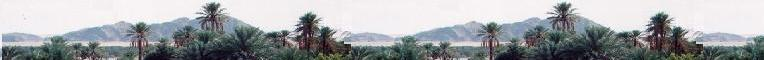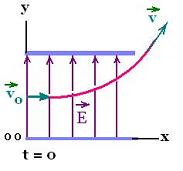Electrostatics

Electromagnetics

Electricity &
Magnetism

# Electrostatics: Effect of the field on a charged particle.

### 1. Free charged particle in a electric field

We are considering the simplest situation where the electric field is uniform .

In an electric field E, if the only significant force on a charged particle q is the electric force F, then the Coulomb's law and Newton second law together give the expression of the net force:

F = q E = m a or a = q E/m

a is the acceleration of the charged particle of mass m.

1. A charged particle released in a uniform electric field E will be accelerated a long the electric field.

At the origin, we place the release point and orient the field parallel to the x-axis.

The kinematics of the particle released from rest (vo = 0), like a free-fall particle, is:

ax = q E/m

x = (1/2)axt2 = (1/2)(qE/m)t2
vx = axt = (qE/m)t

ax = q(E/m) x = (1/2)axt2 = (1/2)(qE/m)t2

### 2. Projectile charged particle in a electric field

Now if the positive charged particle enters the field E oriented along the y-axis, perpendicularly with an initial velocity vo at t = 0, like a projectile, its kinematics equations are:ax = 0
ay = qE/m
x = vot
vx = vo
vy = ayt = (qE/m)t
x = vot
y = (1/2) ayt2 = (1/2) (qE/m)t2 =
(1/2) (qE/m)(x/vo)2 = (1/2) (qE/m)(1/vo)2. x2

That is the particle follows the parabolic path.

vx = vo
vy = ayt = (qE/m)t
x = vot
y = (1/2) ayt2 = (1/2) (qE/m)t2 =
(1/2) (qE/m)(x/vo)2 = (1/2) (qE/m)(1/vo)2. x2

 chimie labs | Physics and Measurements | Probability & Statistics | Combinatorics - Probability | Chimie | Optics | contact |